Next: 3.10 Simulation Results Up: 3. Diffusion Phenomena in Previous: 3.8 Numerical Handling of

# 3.9 Dosis Conservation

For the diffusion simulation applied mesh has to fullfil several physical and mathematical based requirements. Diffusion simulation follows in general after ion implatantation simulation and therefore first requirement is that concentration dosis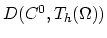calculated on mesh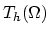has to have approximately the same value as implanted dosis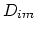. When we take some demanded tolerance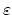, it must also hold,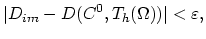(3.143)

thereby, the dosis contained in the simulation mesh is calculated as,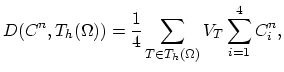(3.144)

and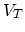is the volume of the element. Furthermore, the chosen mesh and numerical procedure has to ensure accuracy and stability of the obtained solution for each discrete time step. This requirement is expressed by,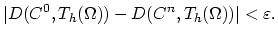(3.145)

The dosis conservation conditions (3.141) and (3.142) are of primarily importance in this work. In order to study the obtained numerical solutions for the given mesh the error estimators presented in Section 2.9 are also used.Next: 3.10 Simulation Results Up: 3. Diffusion Phenomena in Previous: 3.8 Numerical Handling of

H. Ceric: Numerical Techniques in Modern TCAD